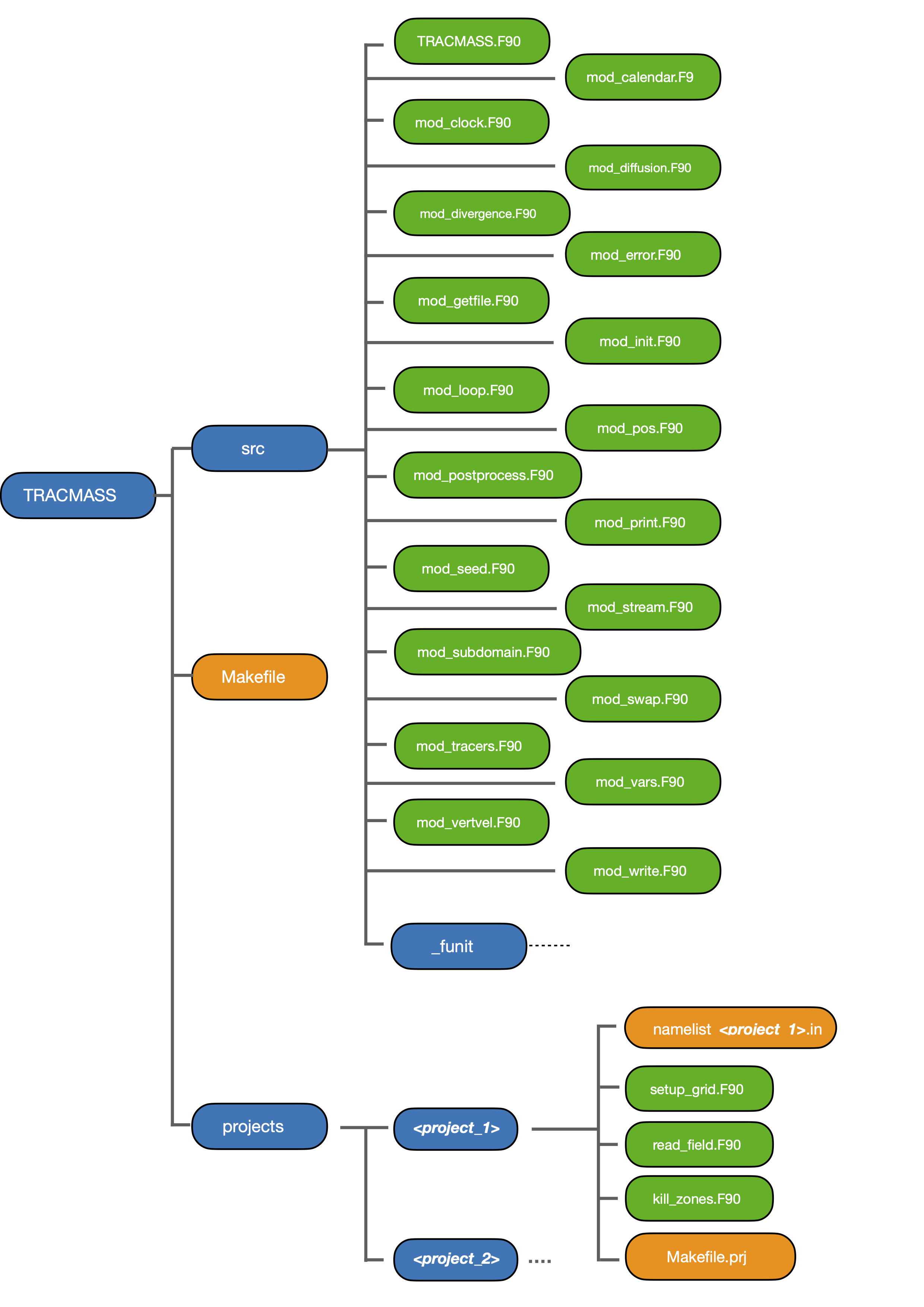The present chapter describes the main program TRACMASS and the different running options.

## TRACMASS.F90

This is how the TRACMASS main program works:

1 - First the namelist is read (init_namelist) and the tracers are initialised (init_tracer).

2 - Then the subdomain is defined (init_subdomain) and all the arrays are allocated (init_alloc).

3 - If norun is false the main subroutines to compute trajectories are called.

3.1 - The grid variables are defined (setup_grid),the calendar (init_calendar) and the seeding are initialised (init_seed).

3.2 - If online stream function are activated, the fluxes are allocated (init_stream).

3.3 - The main loop is called where the trajectories are computed (loop).

4 - Then, the output is postprocessed.

4.1 - If a summary is computed the the subroutine postprocessing is called.

4.2 - If only offline streamfunctions are computed (no summary), the subroutine compute_stream is called.

## ./runtracmass running arguments

TRACMASS can be run with different arguments that can be combined:

• rerun: rerun TRACMASS by seeding only the trajectories that are stored in the rerun file. The model will crash if no rerun file can be found.

• norun: run TRACMASS without the computation of trajectories. This argument is useful to compute offline stream functions from output files.

• summary: include a more detailed summary of the trajectories and their transport at the end of the run.

## TRACMASS unix file tree